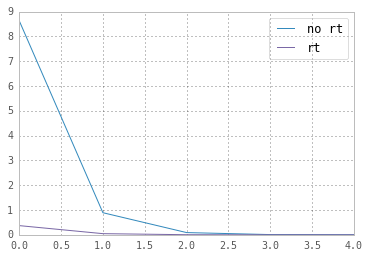# Reparameterization Trick¶

Here we will understand the reparameterization trick used by Kingma and Welling (2014) to train their variational autoencoder.

Assume we have a normal distribution $q$ that is parameterized by $\theta$, specifically $q_{\theta}(x) = N(\theta,1)$. We want to solve the below problem $$\text{min}_{\theta} \quad E_q[x^2]$$ This is of course a rather silly problem and the optimal $\theta$ is obvious. We want to understand how the reparameterization trick helps in calculating the gradient of this objective $E_q[x^2]$.

One way to calculate $\nabla_{\theta} E_q[x^2]$ is as follows $$\nabla_{\theta} E_q[x^2] = \nabla_{\theta} \int q_{\theta}(x) x^2 dx = \int x^2 \nabla_{\theta} q_{\theta}(x) \frac{q_{\theta}(x)}{q_{\theta}(x)} dx = \int q_{\theta}(x) \nabla_{\theta} \log q_{\theta}(x) x^2 dx = E_q[x^2 \nabla_{\theta} \log q_{\theta}(x)]$$

For our example where $q_{\theta}(x) = N(\theta,1)$, this method gives $$\nabla_{\theta} E_q[x^2] = E_q[x^2 (x-\theta)]$$

Reparameterization trick is a way to rewrite the expectation so that the distribution with respect to which we take the expectation is independent of parameter $\theta$. To achieve this, we need to make the stochastic element in $q$ independent of $\theta$. Hence, we write $x$ as $$x = \theta + \epsilon, \quad \epsilon \sim N(0,1)$$ Then, we can write $$E_q[x^2] = E_p[(\theta+\epsilon)^2]$$ where $p$ is the distribution of $\epsilon$, i.e., $N(0,1)$. Now we can write the derivative of $E_q[x^2]$ as follows $$\nabla_{\theta} E_q[x^2] = \nabla_{\theta} E_p[(\theta+\epsilon)^2] = E_p[2(\theta+\epsilon)]$$

Now let us compare the variances of the two methods; we are hoping to see that the first method has high variance while reparameterization trick decreases the variance substantially.

In :
import numpy as np
N = 1000
theta = 2.0
eps = np.random.randn(N)
x = theta + eps

grad1 = lambda x: np.sum(np.square(x)*(x-theta)) / x.size
grad2 = lambda eps: np.sum(2*(theta + eps)) / x.size


3.86872102149
4.03506045463


Let us plot the variance for different sample sizes.

In :
Ns = [10, 100, 1000, 10000, 100000]
reps = 100

means1 = np.zeros(len(Ns))
vars1 = np.zeros(len(Ns))
means2 = np.zeros(len(Ns))
vars2 = np.zeros(len(Ns))

est1 = np.zeros(reps)
est2 = np.zeros(reps)
for i, N in enumerate(Ns):
for r in range(reps):
x = np.random.randn(N) + theta
eps = np.random.randn(N)
means1[i] = np.mean(est1)
means2[i] = np.mean(est2)
vars1[i] = np.var(est1)
vars2[i] = np.var(est2)

print means1
print means2
print
print vars1
print vars2

[ 4.10377908  4.07894165  3.97133622  4.00847457  3.99620013]
[ 3.95374031  4.0025519   3.99285189  4.00065614  4.00154934]

[  8.63411090e+00   8.90650401e-01   8.94014392e-02   8.95798809e-03
1.09726802e-03]
[  3.70336929e-01   4.60841910e-02   3.59508788e-03   3.94404543e-04
3.97245142e-05]

In :
%matplotlib inline
import matplotlib.pyplot as plt

plt.plot(vars1)
plt.plot(vars2)
plt.legend(['no rt', 'rt'])

/usr/local/lib/python2.7/dist-packages/matplotlib/__init__.py:872: UserWarning: axes.color_cycle is deprecated and replaced with axes.prop_cycle; please use the latter.
warnings.warn(self.msg_depr % (key, alt_key))

Out:
<matplotlib.legend.Legend at 0x7facb844ae50>Variance of the estimates using reparameterization trick is one order of magnitude smaller than the estimates from the first method!